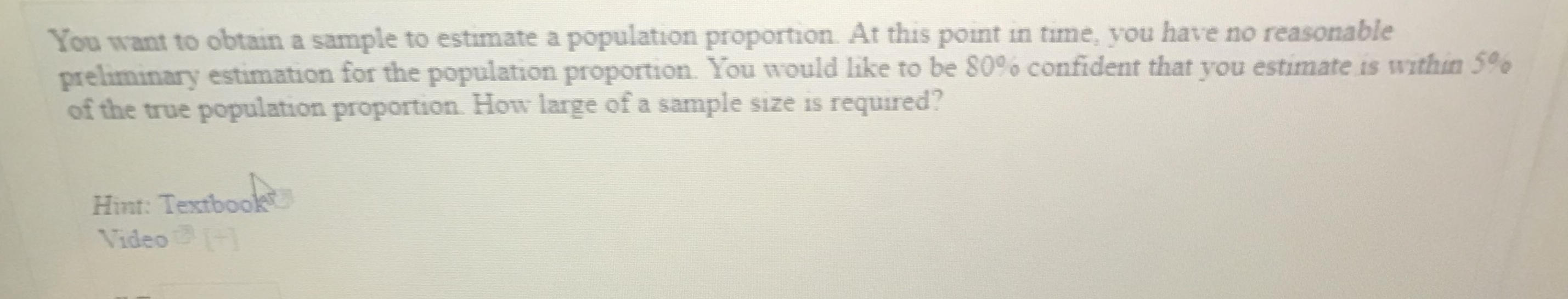# You want to obtain a sample to estimate a population proportion. At this point in time, you have no reasonablepreliminary estimation for the population proportion. You would like to be S0% confident that you estimate is within 5%of the true population proportion. How large of a sample size is required?Hint: TextbooVideo

Questionhelp_outlineImage TranscriptioncloseYou want to obtain a sample to estimate a population proportion. At this point in time, you have no reasonable preliminary estimation for the population proportion. You would like to be S0% confident that you estimate is within 5% of the true population proportion. How large of a sample size is required? Hint: Textboo Video fullscreen
check_circleExpert Solution
Step 1

The exact population proportion is not given here. Therefore the population proportion can be assumed p cap as 0.5.

The confidence level is 80%.

Therefore, the level of significance is 0.20.

The margin of error is given as 0.05.

Step 2

The margin of error is defined as,

Step 3

α = 0.20.

(α/2) = 0.10.

The critical value can be found by the excel formula, =NORM.INV(0....

### Want to see the full answer?

See Solution

#### Want to see this answer and more?

Solutions are written by subject experts who are available 24/7. Questions are typically answered within 1 hour*

See Solution
*Response times may vary by subject and question
Tagged in

### Other